Abdiel Goni — Published On December 23, 2021

This article was published as a part of the Data Science Blogathon

## Optimization

Optimization provides a way to minimize the loss function. Optimization aims to reduce training errors, and Deep Learning Optimization is concerned with finding a suitable model. Another goal of optimization in deep learning is to minimize generalization errors. In this article, we will discuss linear models.

## Linear Model for Regression

The Linear Model is one of the simplest models in machine learning, but linear models are the building blocks for deep neural networks. There are two main classes in supervised learning problems, regression and classification. In reversal, the target value is the actual value. For example, if we have job description data and want to predict how much salary will be given for this job, this is a regression task because the salary is a real value. Or for example, if we have drug stock data and have demand (x1) and usage (x2) variables and the variable to be predicted (dependent) is supply (y). This is also a problem that regression can solve because the inventory variable is a real value.

Whereas if the number of targets is limited, it is a classification task. For example, if we want to recognize an object in an image, say, we want to know if there is a motorcycle, a car, a fence, or perhaps a building or a bicycle in the picture. This is an object recognition task. Since the number of objects is limited, classification can solve this. Or for example, we are analyzing an article and want to know the topic, whether it is about Machine Learning or Computer Vision, or Deep Learning, then that is also a classification task because, once again, the number of target values ​​is limited.

Vector Notation: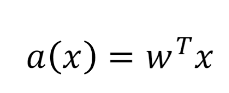For a sample X: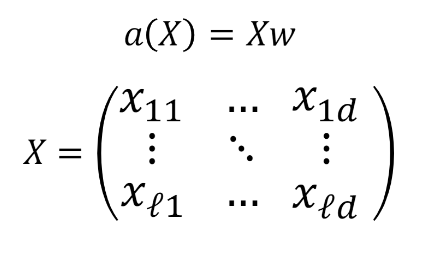This linear model is the point product of the weight vector and the feature vector X., And if we want to apply the model to the entire training set, then we have a Matrix X, which has L rows and d columns. This multiplication produces a vector of size L, and each component predicts the linear model. Then how to measure the model error or know the quality of the train or test set?

Mean Squared Error: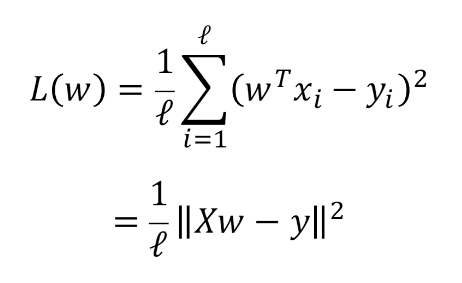The Mean Squared Error is one of the popular choices in regression for the loss function. A particular example, for example, xi. Calculating the model prediction for this example is the product of w and xi, then subtracted from the target value (yi). It then calculates the deviation of the target value from the predicted value, then takes its square and averages the square of the deviation across all training sets. It measures how well our model fits the data. The smaller the mean square error, the better the model provides the data. The mean square error is written in vector form.

Fitting Model: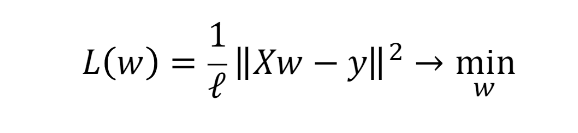The essence of machine learning is optimizing losses to find the best model. So the last function we have, measures how well the model fits the data by minimizing it. So, the goal is to find the set of parameters w that gives this minor mean squared error for the train.

Exact Solution: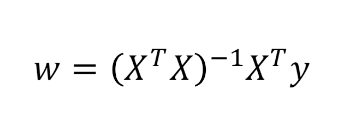If we do the derivative and solve the equation, then we will have an analytical solution to the optimization problem. But it involves inverting and matrices, and highly complex operation and very difficult to find the inverse matrix if it has more features (say more than 100). The linear model for regression is straightforward but valuable (very) for deep neural networks.

## Linear Model for Classification

How to adapt linear methods to classification problems? Logistics Regression. Logistics Regression is a regression model that can be used for classification problems from the simplest classification, namely binary classification, which has only two values ​​on the target (say minus one and one), negative and positive, yes/no, success/failure even with multiple classification problems classes such as strongly agree, agree, strongly disagree and disagree.

Let’s start by solving a 2D classification problem with synthetic data using Python programming to better understand how the Algorithm works.

import numpy as np
from sklearn.datasets import make_moons
from matplotlib import pyplot
from pandas import DataFrame
# generate 2d classification dataset
X, y = make_moons(n_samples=1000, noise=0.5)
# scatter plot, dots colored by class value
df = DataFrame(dict(x=X[:,0], y=X[:,1], label=y))
colors = {0:’red’, 1:’blue’}
fig, ax = pyplot.subplots()
grouped = df.groupby(‘label’)
for key, group in grouped:
group.plot(ax=ax, kind=’scatter’, x=’x’, y=’y’, label=key, color=colors[key])
pyplot.show()

The output of the above code:It cannot be separated linearly. That’s the state of the data above that we can see. Then we have to add features or use a non-linear model. Because the decision line between the two classes is circular, we can add a quadratic part to make the problem in the data above separated linearly, as shown below.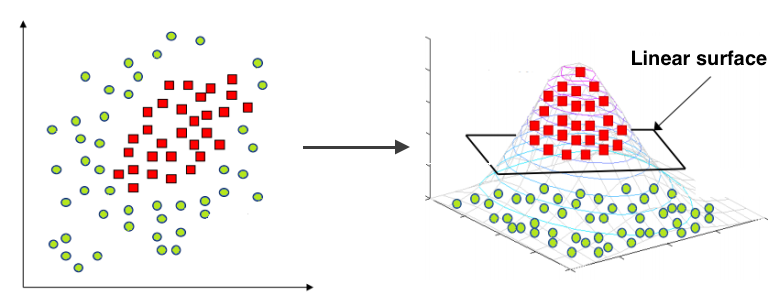The next step is to add features. The expansion allows linear models to make non-linear separations.

```def expand(X):
X_expanded = np.zeros((X.shape, 6))
X_expanded[:, 0] = X[:, 0]
X_expanded[:, 1] = X[:, 1]
X_expanded[:, 2] = X[:, 0] ** 2
X_expanded[:, 3] = X[:, 1] ** 2
X_expanded[:, 4] = X[:, 0] * X[:, 1]
X_expanded[:, 5] = 1
return X_expanded```

The above function works like: For each sample (row in matrix), compute an expanded row: [feature0, feature1, feature0^2, feature1^2, feature0*feature1, 1]

Next, let’s look at the Logistics Regression section. When classifying the thing, we will obtain the probability that the object belongs to class ‘1’. Linear models and logistic functions as below are used to predict chances.

Logistic Function: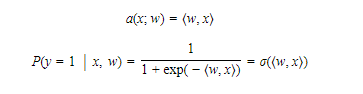```def probability(X, w):
return 1 / (1 + np.exp(-np.dot(X, w)))
dummy_weights = np.linspace(-1, 1, 6)```

predict_prob = probability(X_expanded[:1, :], dummy_weights)

The probability is approximately 0.8678884252629746

Next is to calculate the loss in Logistic Regression with cross-entropy. In logistic regression the optimal parameters w are found by cross-entropy minimization.

Loss for one sample: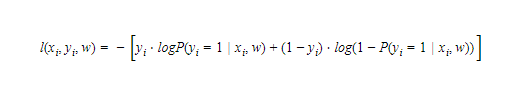Loss for many sample: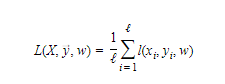To calculate the loss, we use the function:

```def compute_loss(X, y, w):
l = X.shape
p = probability(X, w)
return -(1.0/l) * np.sum(y * np.log(p) + (1-y) * np.log(1-p))
cross_ent = compute_loss(X_expanded, y, dummy_weights)```

The cross-entropy value is about 1.0523716363491382

## Conclusion

In the Regression problem, we discussed a squared error, a loss function, and an analytical solution, but it isn’t easy to calculate. In Logistic Regression, the optimal parameters are found by cross-entropy minimization.